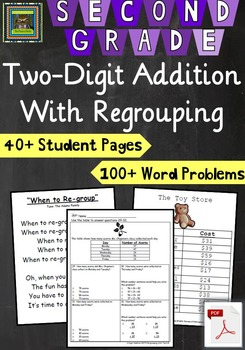Subject
Resource Type
Product Rating
File Type

PDF (Acrobat) Document File

1 MB|45 pages
Share
Product Description
This unit is also available in a combo pack—You get 2 Print and Teach units for \$5.75 that include both the PDF and an editable Word Doc file! Perfect for teaching 2-digit addition WITH and WITHOUT re-grouping! See the product HERE in my TPT store.

DESCRIPTION
Complete print and teach unit for step-by-step direct instruction of 2-digit addition WITH re-grouping. 45 pages of content with over 100 word problems!

Contains everything needed to do guided and independent practice and includes a unit test to check for mastery. Addresses 2nd Grade MATH TEKS for the state of Texas. Designed to prepare students for the level of rigor required to master the STAAR assessment in 3rd grade math. After direct teaching of skills, students move into problem-solving with word problems, data, and graphic sources of information!

*NOW includes lesson guide showing mini-lessons and page numbers
*Could be printed and made into a workbook to fully teach and assess the unit.
*Unit is provided as a PDF for ease of printing.
*Over 100 word problems and 10 word problem starters
*Preview shows EVERYTHING so you can try before you buy, however there are 4 student pages on each page. Product download contains over 40 pages.

WANT MORE SECOND GRADE MATH UNITS AND TESTS?
*2nd Grade Counting Sets of Coins
*2nd Grade Place Value to 999
*2nd Grade Fractions—Parts of a Whole and Set
*2nd Grade Graphs, Data Analysis, and Skip Counting
*Get ALL Pensive Sloth Math Units in a BUNDLE for only \$9.75!

DISCLAIMER
As a Texas teacher, the products I make are designed around the standards for the state of Texas, known as the Texas Essential Knowledge and Skills (TEKS) and are carefully crafted to help students be successful on the Texas STAAR test. I use the rigor of the TEKS and released STAAR assessments as models to guide me when putting together lessons and materials for my students. Thus, I am careful to post products that, in my judgment, address these standards. I am not affiliated in any way with these entities and any and all claims are mine, not those of the groups behind the TEKS or STAAR test.

There are many teachers in Common Core states looking for quality materials. If one of my TEKS based products addresses standards in the Common Core, I will include those standards in this description. I do not teach the Common Core in my classroom, but am familiar with the standards. I am not affiliated with the entity behind Common Core and all claims are my judgment, not those of the groups behind the Common Core.

NEW MATH TEKS (2012)
(1) Mathematical process standards. The student uses mathematical processes to acquire and demonstrate mathematical understanding. The student is expected to:

(A) apply mathematics to problems arising in everyday life, society, and the workplace;

(B) use a problem-solving model that incorporates analyzing given information, formulating a plan or strategy, determining a solution, justifying the solution, and evaluating the problem-solving process and the reasonableness of the solution;

(C) select tools, including real objects, manipulatives, paper and pencil, and technology as appropriate, and techniques, including mental math, estimation, and number sense as appropriate, to solve problems;

(D) communicate mathematical ideas, reasoning, and their implications using multiple representations, including symbols, diagrams, graphs, and language as appropriate;

(E) create and use representations to organize, record, and communicate mathematical ideas;

(F) analyze mathematical relationships to connect and communicate mathematical ideas; and

(G) display, explain, and justify mathematical ideas and arguments using precise mathematical language in written or oral communication.

(4) Number and operations. The student applies mathematical process standards to develop and use strategies and methods for whole number computations in order to solve addition and subtraction problems with efficiency and accuracy. The student is expected to:

(A) recall basic facts to add and subtract within 20 with automaticity;

(B) add up to four two-digit numbers and subtract two-digit numbers using mental strategies and algorithms based on knowledge of place value and properties of operations;

OLD MATH TEKS
(3) Number, operation, and quantitative reasoning. The student adds and subtracts whole numbers to solve problems. The student is expected to:

(B) model addition and subtraction of two-digit numbers with objects, pictures, words, and numbers;

(12) Underlying processes and mathematical tools. The student applies Grade 2 mathematics to solve problems connected to everyday experiences and activities in and outside of school. The student is expected to:

(A) identify the mathematics in everyday situations;

(B) solve problems with guidance that incorporates the processes of understanding the problem, making a plan, carrying out the plan, and evaluating the solution for reasonableness;

(C) select or develop an appropriate problem-solving plan or strategy including drawing a picture, looking for a pattern, systematic guessing and checking, or acting it out in order to solve a problem; and

COMMON CORE
*CCSS.Math.Content.2.OA.A.1 Use addition and subtraction within 100 to solve one- and two-step word problems involving situations of adding to, taking from, putting together, taking apart, and comparing, with unknowns in all positions, e.g., by using drawings and equations with a symbol for the unknown number to represent the problem.

*CCSS.Math.Content.2.NBT.B.5 Fluently add and subtract within 100 using strategies based on place value, properties of operations, and/or the relationship between addition and subtraction.

*CCSS.Math.Content.2.NBT.B.6 Add up to four two-digit numbers using strategies based on place value and properties of operations.

*CCSS.Math.Content.2.MD.D.10 Draw a picture graph and a bar graph (with single-unit scale) to represent a data set with up to four categories. Solve simple put-together, take-apart, and compare problems using information presented in a bar graph.
Total Pages
45 pages